# Existence results of fractional integro-differential equations with m-point multi-term fractional order integral boundary conditions

## Abstract

In this article, we present some new existence and uniqueness results for nonlinear fractional integro-differential equations with m-point multi-term fractional order integral boundary conditions. Our results are based on the Banach contraction principle and Krasnoselskii’s fixed point theorem.

MSC:26A33, 34B15.

## 1 Introduction

Differential equations of fractional order arise in several research areas of science and engineering, such as physics, chemistry, aerodynamics, electro-dynamics of complex medium, polymer rheology, electrical networks, control of dynamical systems, etc. Fractional differential equations provide an excellent tool for description of memory and hereditary properties of various materials and processes. Some recent contributions to the theory of fractional differential equations and its applications can be seen in .

Recently, many researchers have given attention to the existence of solutions of the initial and boundary value problems for fractional differential equations. There are some papers that have studied the existence of solutions to boundary value problems with two-point, three-point, multi-point or integral boundary conditions. See for examples . However, to the best of the authors’ knowledge, there are only a few papers that consider nonlinear fractional differential equations with nonlocal fractional order integral boundary conditions, see .

In this article, we study the existence and uniqueness of solutions for the following nonlinear fractional integro-differential equation with m-point multi-term fractional order integral boundary condition: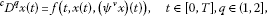(1.1)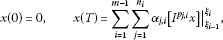(1.2)

where ${}^{c}D^{q}$ denotes the Caputo fractional derivative of order q, $\left[{I}^{{p}_{j,i}}x\right]{|}_{{\xi }_{i-1}}^{{\xi }_{i}}=\left[{I}^{{p}_{j,i}}x\right]\left({\xi }_{i}\right)-\left[{I}^{{p}_{j,i}}x\right]\left({\xi }_{i-1}\right)$, ${I}^{{p}_{j,i}}$ is the Riemann-Liouville fractional integral of order ${p}_{j,i}>0$, $j=1,2,\dots ,{n}_{i}$, ${n}_{i}\in N=\left\{1,2,\dots \right\}$, $i=1,2,\dots ,m-1$, $0={\xi }_{0}<{\xi }_{1}<{\xi }_{2}<\cdots <{\xi }_{m-2}<{\xi }_{m-1}=T$, $f:\left[0,T\right]×X×X\to X$, for $\varphi :\left[0,T\right]×\left[0,T\right]\to \left[0,\mathrm{\infty }\right)$,

$\left({\psi }^{\nu }x\right)\left(t\right)=\left[{I}^{\nu }\varphi x\right]\left(t\right)=\frac{1}{\mathrm{\Gamma }\left(\nu \right)}{\int }_{0}^{t}{\left(t-s\right)}^{\nu -1}\varphi \left(t,s\right)x\left(s\right)\phantom{\rule{0.2em}{0ex}}ds,\phantom{\rule{1em}{0ex}}\nu >0,$

and ${\alpha }_{j,i}\in R$ is such that ${\sum }_{i=1}^{m-1}{\sum }_{j=1}^{{n}_{i}}{\alpha }_{j,i}\left(\frac{{\xi }_{i}^{{p}_{j,i}+1}-{\xi }_{i-1}^{{p}_{j,i}+1}}{\mathrm{\Gamma }\left({p}_{j,i}+2\right)}\right)\ne T$. Let $\left(X,\parallel \cdot \parallel \right)$ be a Banach space and $\mathcal{C}=C\left(\left[0,T\right],X\right)$ denote the Banach space of all continuous functions from $\left[0,T\right]\to X$ endowed with a topology of uniform convergence with the norm denoted by ${\parallel \cdot \parallel }_{\mathcal{C}}$.

We note that if $m=2$, then condition (1.2) reduces to the two-point multi-term fractional integral boundary condition

$x\left(0\right)=0,\phantom{\rule{2em}{0ex}}x\left(T\right)=\sum _{j=1}^{{n}_{1}}{\alpha }_{j,1}\left[{I}^{{p}_{j,1}}x\right]\left(T\right),\phantom{\rule{1em}{0ex}}{n}_{1}\in N=\left\{1,2,\dots \right\}.$

If ${n}_{i}=1$, $i=1,2,\dots ,m-1$, then condition (1.2) reduces to the m-point fractional integral boundary condition

$x\left(0\right)=0,\phantom{\rule{2em}{0ex}}x\left(T\right)=\sum _{i=1}^{m-1}{\alpha }_{1,i}\left[{I}^{{p}_{1,i}}x\right]{|}_{{\xi }_{i-1}}^{{\xi }_{i}}.$
(1.3)

Moreover, if ${p}_{1,i}=1$ and ${\alpha }_{1,i}={\alpha }_{i}$ for $i=1,2,\dots ,m-1$, then condition (1.3) reduces to the usual m-point integral boundary condition

$x\left(0\right)=0,\phantom{\rule{2em}{0ex}}x\left(T\right)={\alpha }_{1}{\int }_{0}^{{\xi }_{1}}x\left(s\right)\phantom{\rule{0.2em}{0ex}}ds+{\alpha }_{2}{\int }_{{\xi }_{1}}^{{\xi }_{2}}x\left(s\right)\phantom{\rule{0.2em}{0ex}}ds+\cdots +{\alpha }_{m-1}{\int }_{{\xi }_{m-2}}^{T}x\left(s\right)\phantom{\rule{0.2em}{0ex}}ds.$

In this case, the boundary condition corresponds to $m-1$ intervals of area under the curve of solution $x\left(t\right)$ from $t={\xi }_{i-1}$ to $t={\xi }_{i}$ for $i=1,2,\dots ,m-1$.

## 2 Preliminaries

Let us recall some basic definitions [2, 4].

Definition 2.1 For a continuous function $f:\left[0,\mathrm{\infty }\right)\to \mathbb{R}$, the Caputo derivative of fractional order q is defined as

${}^{c}D^{q}f\left(t\right)=\frac{1}{\mathrm{\Gamma }\left(n-q\right)}{\int }_{0}^{t}{\left(t-s\right)}^{n-q-1}{f}^{\left(n\right)}\left(s\right)\phantom{\rule{0.2em}{0ex}}ds,\phantom{\rule{1em}{0ex}}n-1

provided that ${f}^{\left(n\right)}\left(t\right)$ exists, where $\left[q\right]$ denotes the integer part of the real number q.

Definition 2.2 The Riemann-Liouville fractional integral of order q for a continuous function $f\left(t\right)$ is defined as

${I}^{q}f\left(t\right)=\frac{1}{\mathrm{\Gamma }\left(q\right)}{\int }_{0}^{t}{\left(t-s\right)}^{q-1}f\left(s\right)\phantom{\rule{0.2em}{0ex}}ds,\phantom{\rule{1em}{0ex}}q>0,$

provided that such integral exists.

Definition 2.3 The Riemann-Liouville fractional derivative of order q for a continuous function $f\left(t\right)$ is defined by

${D}^{q}f\left(t\right)=\frac{1}{\mathrm{\Gamma }\left(n-q\right)}{\left(\frac{d}{dt}\right)}^{n}{\int }_{0}^{t}{\left(t-s\right)}^{n-q-1}f\left(s\right)\phantom{\rule{0.2em}{0ex}}ds,\phantom{\rule{1em}{0ex}}n=\left[q\right]+1,$

provided the right-hand side is pointwise defined on $\left(0,\mathrm{\infty }\right)$.

Lemma 2.1 (see )

Let $q>0$, the general solution of the fractional differential equation

${}^{c}D^{q}x\left(t\right)=0$

has the solution

$x\left(t\right)={k}_{0}+{k}_{1}t+{k}_{2}{t}^{2}+\cdots +{k}_{n-1}{t}^{n-1},$

where ${k}_{i}\in \mathbb{R}$, $i=0,1,2,\dots ,n-1$ ($n=\left[q\right]+1$).

In view of Lemma 2.1, it follows that

${I}^{q}{\phantom{\rule{0.2em}{0ex}}}^{c}{D}^{q}x\left(t\right)=x\left(t\right)+{k}_{0}+{k}_{1}t+{k}_{2}{t}^{2}+\cdots +{k}_{n-1}{t}^{n-1},$
(2.1)

for some ${k}_{i}\in \mathbb{R}$, $i=0,1,2,\dots ,n-1$ ($n=\left[q\right]+1$).

We state a result due to Krasnoselskii  which is needed to prove the existence of at least one solution of the problem (1.1)-(1.2).

Theorem 2.2 Let M be a closed convex and nonempty subset of a Banach space X. Let A, B be operators such that

1. (i)

$Ax+By\in M$ whenever $x,y\in M$,

2. (ii)

A is compact and continuous,

3. (iii)

B is a contraction mapping.

Then there exists $z\in M$ such that $z=Az+Bz$.

Lemma 2.3 Let ${\sum }_{i=1}^{m-1}{\sum }_{j=1}^{{n}_{i}}{\alpha }_{j,i}\left(\frac{{\xi }_{i}^{{p}_{j,i}+1}-{\xi }_{i-1}^{{p}_{j,i}+1}}{\mathrm{\Gamma }\left({p}_{j,i}+2\right)}\right)\ne T$, $1. Then for $h\in C\left[0,T\right]$, the problem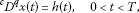(2.2)(2.3)

has a unique solution

$\begin{array}{rcl}x\left(t\right)& =& \frac{1}{\mathrm{\Gamma }\left(q\right)}{\int }_{0}^{t}{\left(t-s\right)}^{q-1}h\left(s\right)\phantom{\rule{0.2em}{0ex}}ds\\ -\frac{t}{\mathrm{\Gamma }\left(q\right)\left(T-{\sum }_{i=1}^{m-1}{\sum }_{j=1}^{{n}_{i}}{\alpha }_{j,i}\frac{{\xi }_{i}^{{p}_{j,i}+1}-{\xi }_{i-1}^{{p}_{j,i}+1}}{\mathrm{\Gamma }\left({p}_{j,i}+2\right)}\right)}{\int }_{0}^{T}{\left(T-s\right)}^{q-1}h\left(s\right)\phantom{\rule{0.2em}{0ex}}ds\\ +\frac{t}{\mathrm{\Gamma }\left(q\right)\left(T-{\sum }_{i=1}^{m-1}{\sum }_{j=1}^{{n}_{i}}{\alpha }_{j,i}\frac{{\xi }_{i}^{{p}_{j,i}+1}-{\xi }_{i-1}^{{p}_{j,i}+1}}{\mathrm{\Gamma }\left({p}_{j,i}+2\right)}\right)}\sum _{i=1}^{m-1}\sum _{j=1}^{{n}_{i}}\frac{{\alpha }_{j,i}}{\mathrm{\Gamma }\left({p}_{j,i}\right)}\\ ×\left[{\int }_{0}^{{\xi }_{i}}{\int }_{0}^{s}{\left({\xi }_{i}-s\right)}^{{p}_{j,i}-1}{\left(s-r\right)}^{q-1}h\left(r\right)\phantom{\rule{0.2em}{0ex}}dr\phantom{\rule{0.2em}{0ex}}ds\\ -{\int }_{0}^{{\xi }_{i-1}}{\int }_{0}^{s}{\left({\xi }_{i-1}-s\right)}^{{p}_{j,i}-1}{\left(s-r\right)}^{q-1}h\left(r\right)\phantom{\rule{0.2em}{0ex}}dr\phantom{\rule{0.2em}{0ex}}ds\right].\end{array}$
(2.4)

Proof We may apply (2.1) to reduce (2.2) to an equivalent integral equation

$x\left(t\right)=\frac{1}{\mathrm{\Gamma }\left(q\right)}{\int }_{0}^{t}{\left(t-s\right)}^{q-1}h\left(s\right)\phantom{\rule{0.2em}{0ex}}ds-{c}_{0}-{c}_{1}t,$
(2.5)

for some constants ${c}_{0},{c}_{1}\in \mathbb{R}$.

From $x\left(0\right)=0$, it follows that ${c}_{0}=0$. Taking the Riemann-Liouville fractional integral of order ${p}_{j,i}$ for (2.5), we get

$\begin{array}{rcl}\left[{I}^{{p}_{j,i}}x\right]\left(t\right)& =& \frac{1}{\mathrm{\Gamma }\left({p}_{j,i}\right)}{\int }_{0}^{t}{\left(t-s\right)}^{{p}_{j,i}-1}\left[\frac{1}{\mathrm{\Gamma }\left(q\right)}{\int }_{0}^{s}{\left(s-r\right)}^{q-1}h\left(r\right)\phantom{\rule{0.2em}{0ex}}dr-{c}_{1}s\right]\phantom{\rule{0.2em}{0ex}}ds\\ =& \frac{1}{\mathrm{\Gamma }\left({p}_{j,i}\right)\mathrm{\Gamma }\left(q\right)}{\int }_{0}^{t}{\int }_{0}^{s}{\left(t-s\right)}^{{p}_{j,i}-1}{\left(s-r\right)}^{q-1}h\left(r\right)\phantom{\rule{0.2em}{0ex}}dr\phantom{\rule{0.2em}{0ex}}ds-{c}_{1}\frac{{t}^{{p}_{j,i}+1}}{\mathrm{\Gamma }\left({p}_{j,i}+2\right)}.\end{array}$

On the other hand, for $0\le {\xi }_{i-1}\le {\xi }_{i}\le T$, we obtain

$\begin{array}{rcl}\left[{I}^{{p}_{j,i}}x\right]{|}_{{\xi }_{i-1}}^{{\xi }_{i}}& =& \left[{I}^{{p}_{j,i}}x\right]\left({\xi }_{i}\right)-\left[{I}^{{p}_{j,i}}x\right]\left({\xi }_{i-1}\right)\\ =& \frac{1}{\mathrm{\Gamma }\left({p}_{j,i}\right)\mathrm{\Gamma }\left(q\right)}\left[{\int }_{0}^{{\xi }_{i}}{\int }_{0}^{s}{\left({\xi }_{i}-s\right)}^{{p}_{j,i}-1}{\left(s-r\right)}^{q-1}h\left(r\right)\phantom{\rule{0.2em}{0ex}}dr\phantom{\rule{0.2em}{0ex}}ds\\ -{\int }_{0}^{{\xi }_{i-1}}{\int }_{0}^{s}{\left({\xi }_{i-1}-s\right)}^{{p}_{j,i}-1}{\left(s-r\right)}^{q-1}h\left(r\right)\phantom{\rule{0.2em}{0ex}}dr\phantom{\rule{0.2em}{0ex}}ds\right]\\ -{c}_{1}\left(\frac{{\xi }_{i}^{{p}_{j,i}+1}-{\xi }_{i-1}^{{p}_{j,i}+1}}{\mathrm{\Gamma }\left({p}_{j,i}+2\right)}\right).\end{array}$

The second condition of (2.3) implies that

$\begin{array}{c}\frac{1}{\mathrm{\Gamma }\left(q\right)}\sum _{i=1}^{m-1}\sum _{j=1}^{{n}_{i}}\frac{{\alpha }_{j,i}}{\mathrm{\Gamma }\left({p}_{j,i}\right)}\left[{\int }_{0}^{{\xi }_{i}}{\int }_{0}^{s}{\left({\xi }_{i}-s\right)}^{{p}_{j,i}-1}{\left(s-r\right)}^{q-1}h\left(r\right)\phantom{\rule{0.2em}{0ex}}dr\phantom{\rule{0.2em}{0ex}}ds\hfill \\ \phantom{\rule{2em}{0ex}}-{\int }_{0}^{{\xi }_{i-1}}{\int }_{0}^{s}{\left({\xi }_{i-1}-s\right)}^{{p}_{j,i}-1}{\left(s-r\right)}^{q-1}h\left(r\right)\phantom{\rule{0.2em}{0ex}}dr\phantom{\rule{0.2em}{0ex}}ds\right]\hfill \\ \phantom{\rule{2em}{0ex}}-{c}_{1}\sum _{i=1}^{m-1}\sum _{j=1}^{{n}_{i}}{\alpha }_{j,i}\left(\frac{{\xi }_{i}^{{p}_{j,i}+1}-{\xi }_{i-1}^{{p}_{j,i}+1}}{\mathrm{\Gamma }\left({p}_{j,i}+2\right)}\right)\hfill \\ \phantom{\rule{1em}{0ex}}=\frac{1}{\mathrm{\Gamma }\left(q\right)}{\int }_{0}^{T}{\left(T-s\right)}^{q-1}h\left(s\right)\phantom{\rule{0.2em}{0ex}}ds-{c}_{1}T.\hfill \end{array}$

Thus,

$\begin{array}{rcl}{c}_{1}& =& \frac{1}{\mathrm{\Gamma }\left(q\right)\left(T-{\sum }_{i=1}^{m-1}{\sum }_{j=1}^{{n}_{i}}{\alpha }_{j,i}\frac{{\xi }_{i}^{{p}_{j,i}+1}-{\xi }_{i-1}^{{p}_{j,i}+1}}{\mathrm{\Gamma }\left({p}_{j,i}+2\right)}\right)}{\int }_{0}^{T}{\left(T-s\right)}^{q-1}h\left(s\right)\phantom{\rule{0.2em}{0ex}}ds\\ -\frac{1}{\mathrm{\Gamma }\left(q\right)\left(T-{\sum }_{i=1}^{m-1}{\sum }_{j=1}^{{n}_{i}}{\alpha }_{j,i}\frac{{\xi }_{i}^{{p}_{j,i}+1}-{\xi }_{i-1}^{{p}_{j,i}+1}}{\mathrm{\Gamma }\left({p}_{j,i}+2\right)}\right)}\sum _{i=1}^{m-1}\sum _{j=1}^{{n}_{i}}\frac{{\alpha }_{j,i}}{\mathrm{\Gamma }\left({p}_{j,i}\right)}\\ ×\left[{\int }_{0}^{{\xi }_{i}}{\int }_{0}^{s}{\left({\xi }_{i}-s\right)}^{{p}_{j,i}-1}{\left(s-r\right)}^{q-1}h\left(r\right)\phantom{\rule{0.2em}{0ex}}dr\phantom{\rule{0.2em}{0ex}}ds\\ -{\int }_{0}^{{\xi }_{i-1}}{\int }_{0}^{s}{\left({\xi }_{i-1}-s\right)}^{{p}_{j,i}-1}{\left(s-r\right)}^{q-1}h\left(r\right)\phantom{\rule{0.2em}{0ex}}dr\phantom{\rule{0.2em}{0ex}}ds\right].\end{array}$

Substituting the values of ${c}_{0}$ and ${c}_{1}$ in (2.5), we obtain the solution (2.4). □

In the following, for the sake of convenience, set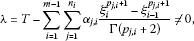(2.6)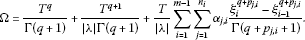(2.7)

We denote $B\left(\cdot ,\cdot \right)$ as the standard beta function such that

$B\left(b+1,a\right)={\int }_{0}^{1}{\left(1-s\right)}^{a-1}{s}^{b}\phantom{\rule{0.2em}{0ex}}ds=\frac{\mathrm{\Gamma }\left(a\right)\mathrm{\Gamma }\left(b+1\right)}{\mathrm{\Gamma }\left(a+b+1\right)}.$

## 3 Main results

Now we are in the position to establish the main result. Our first result is based on Banach’s fixed point theorem.

Theorem 3.1 Assume that $f:\left[0,T\right]×X×X\to X$ is jointly continuous and maps bounded subsets of $\left[0,T\right]×X×X$ in to relatively compact subsets of X, and $\varphi :\left[0,T\right]×\left[0,T\right]\to \left[0,\mathrm{\infty }\right)$ is continuous with ${\varphi }_{0}=max\left\{\varphi \left(t,s\right):\left(t,s\right)\in \left[0,T\right]×\left[0,T\right]\right\}$. In addition, suppose that there exist positive constants ${L}_{1}$, ${L}_{2}$ such that

(${H}_{1}$) $\parallel f\left(t,x\left(t\right),\left({\psi }^{\nu }x\right)\left(t\right)\right)-f\left(t,y\left(t\right),\left({\psi }^{\nu }y\right)\left(t\right)\right)\parallel \le {L}_{1}\parallel x-y\parallel +{L}_{2}\parallel \left({\psi }^{\nu }x\right)-\left({\psi }^{\nu }y\right)\parallel$, for all $t\in \left[0,T\right]$, $x,y\in X$,

(${H}_{2}$) $\mathrm{\Lambda }=:\left({L}_{1}+\frac{{L}_{2}{\varphi }_{0}{T}^{\nu }}{\mathrm{\Gamma }\left(\nu +1\right)}\right)\mathrm{\Omega }<1$, where Ω is defined by (2.7).

Then the problem (1.1)-(1.2) has a unique solution.

Proof We transform the BVP (1.1)-(1.2) into a fixed point problem. In view of Lemma 2.3 and (2.6), we consider the operator $F:\mathcal{C}\to \mathcal{C}$ defined by

$\begin{array}{rcl}\left(Fx\right)\left(t\right)& =& \frac{1}{\mathrm{\Gamma }\left(q\right)}{\int }_{0}^{t}{\left(t-s\right)}^{q-1}f\left(s,x\left(s\right),\left({\psi }^{\nu }x\right)\left(s\right)\right)\phantom{\rule{0.2em}{0ex}}ds\\ -\frac{t}{\lambda \mathrm{\Gamma }\left(q\right)}{\int }_{0}^{T}{\left(T-s\right)}^{q-1}f\left(s,x\left(s\right),\left({\psi }^{\nu }x\right)\left(s\right)\right)\phantom{\rule{0.2em}{0ex}}ds\\ +\frac{t}{\lambda \mathrm{\Gamma }\left(q\right)}\sum _{i=1}^{m-1}\sum _{j=1}^{{n}_{i}}\frac{{\alpha }_{j,i}}{\mathrm{\Gamma }\left({p}_{j,i}\right)}\\ ×\left[{\int }_{0}^{{\xi }_{i}}{\int }_{0}^{s}{\left({\xi }_{i}-s\right)}^{{p}_{j,i}-1}{\left(s-r\right)}^{q-1}f\left(r,x\left(r\right),\left({\psi }^{\nu }x\right)\left(r\right)\right)\phantom{\rule{0.2em}{0ex}}dr\phantom{\rule{0.2em}{0ex}}ds\\ -{\int }_{0}^{{\xi }_{i-1}}{\int }_{0}^{s}{\left({\xi }_{i-1}-s\right)}^{{p}_{j,i}-1}{\left(s-r\right)}^{q-1}f\left(r,x\left(r\right),\left({\psi }^{\nu }x\right)\left(r\right)\right)\phantom{\rule{0.2em}{0ex}}dr\phantom{\rule{0.2em}{0ex}}ds\right],\end{array}$

for $t\in \left[0,T\right]$. By setting ${sup}_{t\in \left[0,T\right]}\parallel f\left(t,0,0\right)\parallel =M$, and choosing

$r\ge \frac{M\mathrm{\Omega }}{1-\mathrm{\Lambda }},$
(3.1)

we will show that $F{B}_{r}\subset {B}_{r}$, where ${B}_{r}=\left\{x\in \mathcal{C}:\parallel x\parallel \le r\right\}$. Then, for $x\in {B}_{r}$, we have

$\begin{array}{rcl}\parallel \left(Fx\right)\left(t\right)\parallel & \le & \frac{1}{\mathrm{\Gamma }\left(q\right)}{\int }_{0}^{t}{\left(t-s\right)}^{q-1}\parallel f\left(s,x\left(s\right),\left({\psi }^{\nu }x\right)\left(s\right)\right)\parallel \phantom{\rule{0.2em}{0ex}}ds\\ +\frac{T}{|\lambda |\mathrm{\Gamma }\left(q\right)}{\int }_{0}^{T}{\left(T-s\right)}^{q-1}\parallel f\left(s,x\left(s\right),\left({\psi }^{\nu }x\right)\left(s\right)\right)\parallel \phantom{\rule{0.2em}{0ex}}ds\\ +\frac{T}{|\lambda |\mathrm{\Gamma }\left(q\right)}\sum _{i=1}^{m-1}\sum _{j=1}^{{n}_{i}}\frac{{\alpha }_{j,i}}{\mathrm{\Gamma }\left({p}_{j,i}\right)}\\ ×\left[{\int }_{0}^{{\xi }_{i}}{\int }_{0}^{s}{\left({\xi }_{i}-s\right)}^{{p}_{j,i}-1}{\left(s-r\right)}^{q-1}\parallel f\left(r,x\left(r\right),\left({\psi }^{\nu }x\right)\left(r\right)\right)\parallel \phantom{\rule{0.2em}{0ex}}dr\phantom{\rule{0.2em}{0ex}}ds\\ -{\int }_{0}^{{\xi }_{i-1}}{\int }_{0}^{s}{\left({\xi }_{i-1}-s\right)}^{{p}_{j,i}-1}{\left(s-r\right)}^{q-1}\parallel f\left(r,x\left(r\right),\left({\psi }^{\nu }x\right)\left(r\right)\right)\parallel \phantom{\rule{0.2em}{0ex}}dr\phantom{\rule{0.2em}{0ex}}ds\right]\\ \le & \frac{1}{\mathrm{\Gamma }\left(q\right)}{\int }_{0}^{t}{\left(t-s\right)}^{q-1}\left(\parallel f\left(s,x\left(s\right),\left({\psi }^{\nu }x\right)\left(s\right)\right)-f\left(s,0,0\right)\parallel +\parallel f\left(s,0,0\right)\parallel \right)\phantom{\rule{0.2em}{0ex}}ds\\ +\frac{T}{|\lambda |\mathrm{\Gamma }\left(q\right)}{\int }_{0}^{T}{\left(T-s\right)}^{q-1}\left(\parallel f\left(s,x\left(s\right),\left({\psi }^{\nu }x\right)\left(s\right)\right)-f\left(s,0,0\right)\parallel +\parallel f\left(s,0,0\right)\parallel \right)\phantom{\rule{0.2em}{0ex}}ds\\ +\frac{T}{|\lambda |\mathrm{\Gamma }\left(q\right)}\sum _{i=1}^{m-1}\sum _{j=1}^{{n}_{i}}\frac{{\alpha }_{j,i}}{\mathrm{\Gamma }\left({p}_{j,i}\right)}\left[{\int }_{0}^{{\xi }_{i}}{\int }_{0}^{s}{\left({\xi }_{i}-s\right)}^{{p}_{j,i}-1}{\left(s-r\right)}^{q-1}\\ ×\left(\parallel f\left(r,x\left(r\right),\left({\psi }^{\nu }x\right)\left(r\right)\right)-f\left(s,0,0\right)\parallel +\parallel f\left(s,0,0\right)\parallel \right)\phantom{\rule{0.2em}{0ex}}dr\phantom{\rule{0.2em}{0ex}}ds\\ -{\int }_{0}^{{\xi }_{i-1}}{\int }_{0}^{s}{\left({\xi }_{i-1}-s\right)}^{{p}_{j,i}-1}{\left(s-r\right)}^{q-1}\\ ×\left(\parallel f\left(r,x\left(r\right),\left({\psi }^{\nu }x\right)\left(r\right)\right)-f\left(s,0,0\right)\parallel +\parallel f\left(s,0,0\right)\parallel \right)\phantom{\rule{0.2em}{0ex}}dr\phantom{\rule{0.2em}{0ex}}ds\right]\\ \le & \frac{1}{\mathrm{\Gamma }\left(q\right)}\left[\left({L}_{1}+\frac{{L}_{2}{\varphi }_{0}{T}^{\nu }}{\mathrm{\Gamma }\left(\nu +1\right)}\right)r+M\right]{\int }_{0}^{t}{\left(t-s\right)}^{q-1}\phantom{\rule{0.2em}{0ex}}ds\\ +\frac{T}{|\lambda |\mathrm{\Gamma }\left(q\right)}\left[\left({L}_{1}+\frac{{L}_{2}{\varphi }_{0}{T}^{\nu }}{\mathrm{\Gamma }\left(\nu +1\right)}\right)r+M\right]{\int }_{0}^{T}{\left(T-s\right)}^{q-1}\phantom{\rule{0.2em}{0ex}}ds\\ +\frac{T}{|\lambda |\mathrm{\Gamma }\left(q\right)}\left[\left({L}_{1}+\frac{{L}_{2}{\varphi }_{0}{T}^{\nu }}{\mathrm{\Gamma }\left(\nu +1\right)}\right)r+M\right]\\ ×\sum _{i=1}^{m-1}\sum _{j=1}^{{n}_{i}}\frac{{\alpha }_{j,i}}{\mathrm{\Gamma }\left({p}_{j,i}\right)}\left[{\int }_{0}^{{\xi }_{i}}{\int }_{0}^{s}{\left({\xi }_{i}-s\right)}^{{p}_{j,i}-1}{\left(s-r\right)}^{q-1}\phantom{\rule{0.2em}{0ex}}dr\phantom{\rule{0.2em}{0ex}}ds\\ -{\int }_{0}^{{\xi }_{i-1}}{\int }_{0}^{s}{\left({\xi }_{i-1}-s\right)}^{{p}_{j,i}-1}{\left(s-r\right)}^{q-1}\phantom{\rule{0.2em}{0ex}}dr\phantom{\rule{0.2em}{0ex}}ds\right]\\ \le & \left(\left({L}_{1}+\frac{{L}_{2}{\varphi }_{0}{T}^{\nu }}{\mathrm{\Gamma }\left(\nu +1\right)}\right)r+M\right)\left[\frac{{T}^{q}}{\mathrm{\Gamma }\left(q+1\right)}+\frac{{T}^{q+1}}{|\lambda |\mathrm{\Gamma }\left(q+1\right)}\\ +\frac{T}{|\lambda |\mathrm{\Gamma }\left(q+1\right)}\sum _{i=1}^{m-1}\sum _{j=1}^{{n}_{i}}\frac{{\alpha }_{j,i}}{\mathrm{\Gamma }\left({p}_{j,i}\right)}\left({\xi }_{i}^{q+{p}_{j,i}}B\left(q+1,{p}_{j,i}\right)-{\xi }_{i-1}^{q+{p}_{j,i}}B\left(q+1,{p}_{j,i}\right)\right)\right]\\ =& \left(\left({L}_{1}+\frac{{L}_{2}{\varphi }_{0}{T}^{\nu }}{\mathrm{\Gamma }\left(\nu +1\right)}\right)r+M\right)\left[\frac{{T}^{q}}{\mathrm{\Gamma }\left(q+1\right)}+\frac{{T}^{q+1}}{|\lambda |\mathrm{\Gamma }\left(q+1\right)}\\ +\frac{T}{|\lambda |}\sum _{i=1}^{m-1}\sum _{j=1}^{{n}_{i}}{\alpha }_{j,i}\frac{{\xi }_{i}^{q+{p}_{j,i}}-{\xi }_{i-1}^{q+{p}_{j,i}}}{\mathrm{\Gamma }\left(q+{p}_{j,i}+1\right)}\right]=\mathrm{\Omega }\left[\left({L}_{1}+\frac{{L}_{2}{\varphi }_{0}{T}^{\nu }}{\mathrm{\Gamma }\left(\nu +1\right)}\right)r+M\right]\le r.\end{array}$

In the following, from (${H}_{1}$) for $x,y\in \mathcal{C}$ and for each $t\in \left[0,T\right]$, we have

$\begin{array}{c}\parallel \left(Fx\right)\left(t\right)-\left(Fy\right)\left(t\right)\parallel \hfill \\ \phantom{\rule{1em}{0ex}}\le \frac{1}{\mathrm{\Gamma }\left(q\right)}{\int }_{0}^{t}{\left(t-s\right)}^{q-1}\parallel f\left(s,x\left(s\right),\left({\psi }^{\nu }x\right)\left(s\right)\right)-f\left(s,y\left(s\right),\left({\psi }^{\nu }y\right)\left(s\right)\right)\parallel \phantom{\rule{0.2em}{0ex}}ds\hfill \\ \phantom{\rule{2em}{0ex}}+\frac{T}{|\lambda |\mathrm{\Gamma }\left(q\right)}{\int }_{0}^{T}{\left(T-s\right)}^{q-1}\parallel f\left(s,x\left(s\right),\left({\psi }^{\nu }x\right)\left(s\right)\right)-f\left(s,y\left(s\right),\left({\psi }^{\nu }y\right)\left(s\right)\right)\parallel \phantom{\rule{0.2em}{0ex}}ds\hfill \\ \phantom{\rule{2em}{0ex}}+\frac{T}{|\lambda |\mathrm{\Gamma }\left(q\right)}\sum _{i=1}^{m-1}\sum _{j=1}^{{n}_{i}}\frac{{\alpha }_{j,i}}{\mathrm{\Gamma }\left({p}_{j,i}\right)}\hfill \\ \phantom{\rule{2em}{0ex}}×\left[{\int }_{0}^{{\xi }_{i}}{\int }_{0}^{s}{\left({\xi }_{i}-s\right)}^{{p}_{j,i}-1}{\left(s-r\right)}^{q-1}\parallel f\left(r,x\left(r\right),\left({\psi }^{\nu }x\right)\left(r\right)\right)-f\left(r,y\left(r\right),\left({\psi }^{\nu }y\right)\left(r\right)\right)\parallel \phantom{\rule{0.2em}{0ex}}dr\phantom{\rule{0.2em}{0ex}}ds\hfill \\ \phantom{\rule{2em}{0ex}}-{\int }_{0}^{{\xi }_{i-1}}{\int }_{0}^{s}{\left({\xi }_{i-1}-s\right)}^{{p}_{j,i}-1}{\left(s-r\right)}^{q-1}\parallel f\left(r,x\left(r\right),\left({\psi }^{\nu }x\right)\left(r\right)\right)-f\left(r,y\left(r\right),\left({\psi }^{\nu }y\right)\left(r\right)\right)\parallel \phantom{\rule{0.2em}{0ex}}dr\phantom{\rule{0.2em}{0ex}}ds\right]\hfill \\ \phantom{\rule{1em}{0ex}}\le \frac{1}{\mathrm{\Gamma }\left(q\right)}\left({L}_{1}+\frac{{L}_{2}{\varphi }_{0}{T}^{\nu }}{\mathrm{\Gamma }\left(\nu +1\right)}\right){\parallel x-y\parallel }_{\mathcal{C}}{\int }_{0}^{t}{\left(t-s\right)}^{q-1}\phantom{\rule{0.2em}{0ex}}ds\hfill \\ \phantom{\rule{2em}{0ex}}+\frac{T}{|\lambda |\mathrm{\Gamma }\left(q\right)}\left({L}_{1}+\frac{{L}_{2}{\varphi }_{0}{T}^{\nu }}{\mathrm{\Gamma }\left(\nu +1\right)}\right){\parallel x-y\parallel }_{\mathcal{C}}{\int }_{0}^{T}{\left(T-s\right)}^{q-1}\phantom{\rule{0.2em}{0ex}}ds\hfill \\ \phantom{\rule{2em}{0ex}}+\frac{T}{|\lambda |\mathrm{\Gamma }\left(q\right)}\left({L}_{1}+\frac{{L}_{2}{\varphi }_{0}{T}^{\nu }}{\mathrm{\Gamma }\left(\nu +1\right)}\right){\parallel x-y\parallel }_{\mathcal{C}}\hfill \\ \phantom{\rule{2em}{0ex}}×\sum _{i=1}^{m-1}\sum _{j=1}^{{n}_{i}}\frac{{\alpha }_{j,i}}{\mathrm{\Gamma }\left({p}_{j,i}\right)}\left[{\int }_{0}^{{\xi }_{i}}{\int }_{0}^{s}{\left({\xi }_{i}-s\right)}^{{p}_{j,i}-1}{\left(s-r\right)}^{q-1}\phantom{\rule{0.2em}{0ex}}dr\phantom{\rule{0.2em}{0ex}}ds\hfill \\ \phantom{\rule{2em}{0ex}}-{\int }_{0}^{{\xi }_{i-1}}{\int }_{0}^{s}{\left({\xi }_{i-1}-s\right)}^{{p}_{j,i}-1}{\left(s-r\right)}^{q-1}\phantom{\rule{0.2em}{0ex}}dr\phantom{\rule{0.2em}{0ex}}ds\right]\hfill \\ \phantom{\rule{1em}{0ex}}\le \left({L}_{1}+\frac{{L}_{2}{\varphi }_{0}{T}^{\nu }}{\mathrm{\Gamma }\left(\nu +1\right)}\right)\mathrm{\Omega }{\parallel x-y\parallel }_{\mathcal{C}}=\mathrm{\Lambda }{\parallel x-y\parallel }_{\mathcal{C}}.\hfill \end{array}$

By (${H}_{2}$), $\mathrm{\Lambda }<1$, therefore, F is a contraction. Hence, by the Banach fixed point theorem, we get that F has a fixed point which is a unique solution of the problem (1.1)-(1.2). □

Our second result is based on Krasnoselskii’s fixed point theorem.

Theorem 3.2 Assume that (${H}_{1}$) and (${H}_{2}$) hold with

$\parallel f\left(t,x\left(t\right),\left({\psi }^{\nu }x\right)\left(t\right)\right)\parallel \le \mu \left(t\right),$
(3.2)

for all $\left(t,x,\left({\psi }^{\nu }x\right)\right)\in \left[0,T\right]×X×X$, where $\mu \in {L}^{1}\left(\left[0,T\right],{R}^{+}\right)$.

Then the BVP (1.1)-(1.2) has at least one solution on $\left[0,T\right]$.

Proof First, we choose

$r\ge {\parallel \mu \parallel }_{{L}^{1}}\mathrm{\Omega },$

where Ω is defined by (2.7), and consider ${B}_{r}=\left\{x\in \mathcal{C}:\parallel x\parallel \le r\right\}$. On ${B}_{r}$ we define the operators S and U as follows: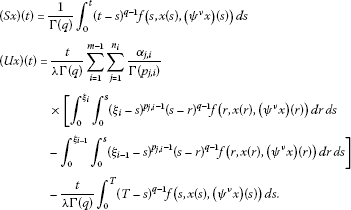Now we shall show that $S+U$ has a fixed point in ${B}_{r}$. For $x,y\in {B}_{r}$, we have

$\begin{array}{rcl}\parallel Sx+Uy\parallel & \le & {\parallel \mu \parallel }_{{L}^{1}}\left(\frac{1}{\mathrm{\Gamma }\left(q\right)}{\int }_{0}^{t}{\left(t-s\right)}^{q-1}\phantom{\rule{0.2em}{0ex}}ds+\frac{T}{|\lambda |\mathrm{\Gamma }\left(q\right)}{\int }_{0}^{T}{\left(T-s\right)}^{q-1}\phantom{\rule{0.2em}{0ex}}ds\\ +\frac{T}{|\lambda |\mathrm{\Gamma }\left(q\right)}\sum _{i=1}^{m-1}\sum _{j=1}^{{n}_{i}}\frac{{\alpha }_{j,i}}{\mathrm{\Gamma }\left({p}_{j,i}\right)}\\ ×\left[{\int }_{0}^{{\xi }_{i}}{\int }_{0}^{s}{\left({\xi }_{i}-s\right)}^{{p}_{j,i}-1}{\left(s-r\right)}^{q-1}\phantom{\rule{0.2em}{0ex}}dr\phantom{\rule{0.2em}{0ex}}ds\\ -{\int }_{0}^{{\xi }_{i-1}}{\int }_{0}^{s}{\left({\xi }_{i-1}-s\right)}^{{p}_{j,i}-1}{\left(s-r\right)}^{q-1}\phantom{\rule{0.2em}{0ex}}dr\phantom{\rule{0.2em}{0ex}}ds\right]\right)\\ \le & {\parallel \mu \parallel }_{{L}^{1}}\mathrm{\Omega }\le r.\end{array}$

Therefore, $Sx+Uy\in {B}_{r}$.

From assumptions (${H}_{1}$) and (${H}_{2}$) for $x,y\in \mathcal{C}$ and for each $t\in \left[0,T\right]$, we get that

$\parallel \left(Ux\right)\left(t\right)-\left(Uy\right)\left(t\right)\parallel \le \mathrm{\Lambda }{\parallel x-y\parallel }_{\mathcal{C}}.$

Hence, U is a contraction mapping.

Next, we show that S is compact and continuous.

The continuity of f implies that the operator S is continuous. By using condition (3.2), we have that S is uniformly bounded on ${B}_{r}$ as

$\parallel Sx\parallel \le \frac{{\parallel \mu \parallel }_{{L}^{1}}{T}^{q}}{\mathrm{\Gamma }\left(q+1\right)}.$

Furthermore, in view of (${H}_{1}$), we define ${sup}_{\left(t,x,\left({\psi }^{\nu }x\right)\right)\in \left[0,T\right]×{B}_{r}×{B}_{r}}\parallel f\left(t,x,\left({\psi }^{\nu }x\right)\right)\parallel =\overline{f}$, and consequently we get

$\begin{array}{c}\parallel \left(Sx\right)\left({t}_{2}\right)-\left(Sx\right)\left({t}_{1}\right)\parallel \hfill \\ \phantom{\rule{1em}{0ex}}=\parallel \frac{1}{\mathrm{\Gamma }\left(q\right)}{\int }_{0}^{{t}_{2}}{\left({t}_{2}-s\right)}^{q-1}f\left(s,x\left(s\right),\left({\psi }^{\nu }x\right)\left(s\right)\right)\phantom{\rule{0.2em}{0ex}}ds\hfill \\ \phantom{\rule{2em}{0ex}}-\frac{1}{\mathrm{\Gamma }\left(q\right)}{\int }_{0}^{{t}_{1}}{\left({t}_{1}-s\right)}^{q-1}f\left(s,x\left(s\right),\left({\psi }^{\nu }x\right)\left(s\right)\right)\phantom{\rule{0.2em}{0ex}}ds\parallel \hfill \\ \phantom{\rule{1em}{0ex}}\le \frac{1}{\mathrm{\Gamma }\left(q\right)}{\int }_{0}^{{t}_{1}}\left({\left({t}_{2}-s\right)}^{q-1}-{\left({t}_{1}-s\right)}^{q-1}\right)\parallel f\left(s,x\left(s\right),\left({\psi }^{\nu }x\right)\left(s\right)\right)\parallel \phantom{\rule{0.2em}{0ex}}ds\hfill \\ \phantom{\rule{2em}{0ex}}+\frac{1}{\mathrm{\Gamma }\left(q\right)}{\int }_{{t}_{1}}^{{t}_{2}}{\left({t}_{2}-s\right)}^{q-1}\parallel f\left(s,x\left(s\right),\left({\psi }^{\nu }x\right)\left(s\right)\right)\parallel \phantom{\rule{0.2em}{0ex}}ds\hfill \\ \phantom{\rule{1em}{0ex}}\le \frac{\overline{f}}{\mathrm{\Gamma }\left(q+1\right)}|2{\left({t}_{2}-{t}_{1}\right)}^{q}+{t}_{2}^{q}-{t}_{1}^{q}|,\hfill \end{array}$

which is independent of x. As ${t}_{2}\to {t}_{1}$, the right-hand side of the above inequality tends to zero. So, S is relatively compact on ${B}_{r}$. Hence, by Arzelá-Ascoli theorem, S is compact on ${B}_{r}$. Thus, all the assumptions of Theorem 2.2 are satisfied. As a consequence of Theorem 2.2, we have that the boundary value problem (1.1)-(1.2) has at least one solution on $\left[0,T\right]$. This completes the proof. □

## 4 An example

Example 4.1 Consider the following four-point multi-term fractional integral boundary value problem: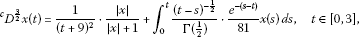(4.1)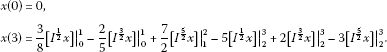(4.2)

Set $q=3/2$, $m=4$, ${\xi }_{0}=0$, ${\xi }_{1}=1$, ${\xi }_{2}=2$, ${\xi }_{3}=T=3$, ${n}_{1}=2$, ${p}_{1,1}=1/2$, ${p}_{2,1}=3/2$, ${\alpha }_{1,1}=3/8$, ${\alpha }_{2,1}=-2/5$, ${n}_{2}=1$, ${p}_{1,2}=5/2$, ${\alpha }_{1,2}=7/2$, ${n}_{3}=3$, ${p}_{1,3}=1/2$, ${p}_{2,3}=3/2$, ${p}_{3,3}=5/2$, ${\alpha }_{1,3}=-5$, ${\alpha }_{2,3}=2$, ${\alpha }_{3,3}=-3$, $\nu =1/2$, $\varphi \left(t,s\right)={e}^{-\left(s-t\right)}/81$, $f\left(t,x\right)=\left(1/{\left(t+9\right)}^{2}\right)\left(|x|/\left(|x|+1\right)\right)+\left({\psi }^{\frac{1}{2}}x\right)\left(t\right)$. Since $\parallel f\left(t,x,\left({\psi }^{\frac{1}{2}}x\right)\right)-f\left(t,y,\left({\psi }^{\frac{1}{2}}y\right)\right)\parallel \le \left(1/81\right)\parallel x-y\parallel +\parallel \left({\psi }^{\frac{1}{2}}x\right)-\left({\psi }^{\frac{1}{2}}y\right)\parallel$, then (${H}_{1}$) and (${H}_{2}$) are satisfied with

$\begin{array}{c}\lambda =T-\sum _{i=1}^{m-1}\sum _{j=1}^{{n}_{i}}{\alpha }_{j,i}\frac{{\xi }_{i}^{{p}_{j,i}+1}-{\xi }_{i-1}^{{p}_{j,i}+1}}{\mathrm{\Gamma }\left({p}_{j,i}+2\right)}\approx 11.80716\ne 0,\hfill \\ \mathrm{\Omega }=\frac{{T}^{q}}{\mathrm{\Gamma }\left(q+1\right)}+\frac{{T}^{q+1}}{|\lambda |\mathrm{\Gamma }\left(q+1\right)}+\frac{T}{|\lambda |}\sum _{i=1}^{m-1}\sum _{j=1}^{{n}_{i}}{\alpha }_{j,i}\frac{{\xi }_{i}^{q+{p}_{j,i}}-{\xi }_{i-1}^{q+{p}_{j,i}}}{\mathrm{\Gamma }\left(q+{p}_{j,i}+1\right)}\approx 1.85722,\hfill \end{array}$

${L}_{1}=1/81$, ${L}_{2}=1$, ${\varphi }_{0}={e}^{3}/81$, ${T}^{\nu }=\sqrt{3}$, $\mathrm{\Gamma }\left(\nu +1\right)=\sqrt{\pi }/2$. We can show that

$\mathrm{\Lambda }=\left({L}_{1}+\frac{{L}_{2}{\varphi }_{0}{T}^{\nu }}{\mathrm{\Gamma }\left(\nu +1\right)}\right)\mathrm{\Omega }\approx 0.92300<1.$

Hence, by Theorem 3.1, the boundary value problem (4.1)-(4.2) has a unique solution on $\left[0,3\right]$.

## References

1. Hilfer R: Fractional Calculus in Physics. World Scientific, Singapore; 2000.

2. Kilbas AA, Srivastava HM, Trujillo JJ: Theory and Applications of Fractional Differential Equations. Elsevier, Amsterdam; 2006.

3. Mainardi F: Fractional Calculus and Waves in Linear Viscoelasticity: An Introduction to Mathematical Models. Imperial College Press, Singapore; 2010.

4. Podlubny I: Fractional Differential Equations. Academic Press, New York; 1999.

5. Sabatier J, Agrawal OP, Machado JAT (Eds): Advances in Fractional Calculus: Theoretical Developments and Applications in Physics and Engineering. Springer, Dordrecht; 2007.

6. Agarwal RP, Ahmad B: Existence theory for anti-periodic boundary value problems of fractional differential equations and inclusions. Comput. Math. Appl. 2011, 62: 1200-1214. 10.1016/j.camwa.2011.03.001

7. Ahmad B, Nieto JJ: Existence results for nonlinear boundary value problems of fractional integrodifferential equations with integral boundary conditions. Bound. Value Probl. 2009., 2009: Article ID 708576

8. Ahmad B, Nieto JJ: Solvability of nonlinear Langevin equation involving two fractional orders with Dirichlet boundary conditions. Int. J. Differ. Equ. 2010., 2010: Article ID 649486

9. Ahmad B, Nieto JJ, Alsaedi A, El-Shahed M: A study of nonlinear Langevin equation involving two fractional orders in different intervals. Nonlinear Anal., Real World Appl. 2012, 13: 599-606. 10.1016/j.nonrwa.2011.07.052

10. Ahmad B, Ntouyas SK, Alsaedi A: New existence results for nonlinear fractional differential equations with three-point integral boundary conditions. Adv. Differ. Equ. 2011., 2011: Article ID 107384

11. Ahmad B, Sivasundaram S: On four-point nonlocal boundary value problems of nonlinear integro-differential equations of fractional order. Appl. Math. Comput. 2010, 217: 480-487. 10.1016/j.amc.2010.05.080

12. Ahmad B, Sivasundaram S: Theory of fractional differential equations with three-point boundary conditions. Commun. Appl. Anal. 2008, 12: 479-484.

13. Bai Z, Qiu T: Existence of positive solution for singular fractional differential equation. Appl. Math. Comput. 2009, 215: 2761-2767. 10.1016/j.amc.2009.09.017

14. Benchohra M, Hamani S, Ntouyas SK: Boundary value problems for differential equations with fractional order. Surv. Math. Appl. 2008, 3: 1-12.

15. Benchohra M, Hamani S, Ntouyas SK: Boundary value problems for differential equations with fractional order and nonlocal conditions. Nonlinear Anal. 2009, 71: 2391-2396. 10.1016/j.na.2009.01.073

16. Cabada A, Wang G: Positive solutions of nonlinear fractional differential equations with integral boundary value conditions. J. Math. Anal. Appl. 2012, 389: 403-411. 10.1016/j.jmaa.2011.11.065

17. Chai G: Existence results for boundary value problems of nonlinear fractional differential equations. Comput. Math. Appl. 2011, 62: 2374-2380. 10.1016/j.camwa.2011.07.025

18. Cui Y: Existence results for singular boundary value problem of nonlinear fractional differential equation. Abstr. Appl. Anal. 2011., 2011: Article ID 605614

19. Hamani S, Benchohra M, Graef JR: Existence results for boundary-value problems with nonlinear fractional differential inclusions and integral conditions. Electron. J. Differ. Equ. 2010, 2010(20):1-16.

20. Jia M, Liu X: Three nonnegative solutions for fractional differential equations with integral boundary conditions. Comput. Math. Appl. 2011, 62: 1405-1412. 10.1016/j.camwa.2011.03.026

21. Liu X, Jia M: Multiple solutions for fractional differential equations with nonlinear boundary conditions. Comput. Math. Appl. 2010, 59: 2880-2886. 10.1016/j.camwa.2010.02.005

22. Nyamoradi N: Existence of solutions for multi point boundary value problems for fractional differential equations. Arab J. Math. Sci. 2012, 18: 165-175. 10.1016/j.ajmsc.2012.01.002

23. Rehman MU, Khan RA: Existence and uniqueness of solutions for multi-point boundary value problems for fractional differential equations. Appl. Math. Lett. 2010, 23: 1038-1044. 10.1016/j.aml.2010.04.033

24. Rehman MU, Khan RA, Asif NA: Three point boundary value problems for nonlinear fractional differential equations. Acta Math. Sci. 2011, 31: 1337-1346.

25. Su X, Zhang S: Solutions to boundary-value problems for nonlinear differential equations of fractional order. Electron. J. Differ. Equ. 2009, 2009(26):1-15.

26. Tian Y, Zhou Y: Positive solutions for multipoint boundary value problem of fractional differential equations. J. Appl. Math. Comput. 2012, 38: 417-427. 10.1007/s12190-011-0487-y

27. Wang Y, Liu L, Wu Y: Positive solutions for a nonlocal fractional differential equation. Nonlinear Anal. 2011, 74: 3599-3605. 10.1016/j.na.2011.02.043

28. Zhao Y, Sun S, Han Z, Li Q: The existence of multiple positive solutions for boundary value problems of nonlinear fractional differential equations. Commun. Nonlinear Sci. Numer. Simul. 2011, 16: 2086-2097. 10.1016/j.cnsns.2010.08.017

29. Zhong W, Lin W: Nonlocal and multi-point boundary value problem for fractional differential equations. Comput. Math. Appl. 2010, 59: 1345-1351. 10.1016/j.camwa.2009.06.032

30. Zhou WX, Chu YD: Existence of solutions for fractional differential equations with multi-point boundary conditions. Commun. Nonlinear Sci. Numer. Simul. 2012, 17: 1142-1148. 10.1016/j.cnsns.2011.07.019

31. Ahmad B, Nieto JJ: Riemann-Liouville fractional integro-differential equations with fractional nonlocal integral boundary conditions. Bound. Value Probl. 2011., 2011: Article ID 36

32. Sudsutad W, Tariboon J: Boundary value problems for fractional differential equations with three-point fractional integral boundary conditions. Adv. Differ. Equ. 2012., 2012: Article ID 93

33. Wang G, Liu W, Ren C: Existence of solutions for multi-point nonlinear differential equations of fractional orders with integral boundary conditions. Electron. J. Differ. Equ. 2012, 2012(54):1-10.

34. Krasnoselskii MA: Two remarks on the method of successive approximations. Usp. Mat. Nauk 1955, 10: 123-127.

## Acknowledgements

This research is supported by the Centre of Excellence in Mathematics, the Commission on Higher Education, Thailand.

## Author information

Authors

### Competing interests

The authors declare that they have no competing interests.

### Authors’ contributions

All authors contributed equally in this paper. They read and approved the final manuscript.

## Rights and permissions

Reprints and Permissions

Sudsutad, W., Tariboon, J. Existence results of fractional integro-differential equations with m-point multi-term fractional order integral boundary conditions. Bound Value Probl 2012, 94 (2012). https://doi.org/10.1186/1687-2770-2012-94